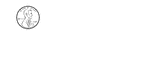1 Cent

Groups of change with totals from 1 to 100 cents using the least amount of coins.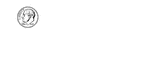10 Cents

Groups of change with totals from 1 to 100 cents using the least amount of coins.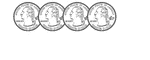100 Cents

Groups of change with totals from 1 to 100 cents using the least amount of coins.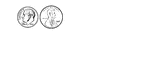11 Cents

Groups of change with totals from 1 to 100 cents using the least amount of coins.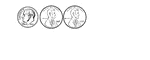12 Cents

Groups of change with totals from 1 to 100 cents using the least amount of coins.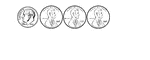13 Cents

Groups of change with totals from 1 to 100 cents using the least amount of coins.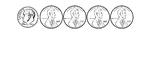14 Cents

Groups of change with totals from 1 to 100 cents using the least amount of coins.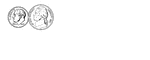15 Cents

Groups of change with totals from 1 to 100 cents using the least amount of coins.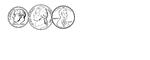16 Cents

Groups of change with totals from 1 to 100 cents using the least amount of coins.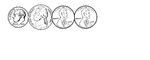17 Cents

Groups of change with totals from 1 to 100 cents using the least amount of coins.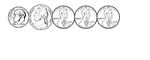18 Cents

Groups of change with totals from 1 to 100 cents using the least amount of coins.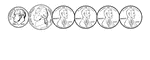19 Cents

Groups of change with totals from 1 to 100 cents using the least amount of coins.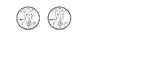2 Cents

Groups of change with totals from 1 to 100 cents using the least amount of coins.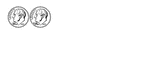20 Cents

Groups of change with totals from 1 to 100 cents using the least amount of coins.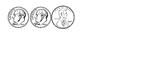21 Cents

Groups of change with totals from 1 to 100 cents using the least amount of coins.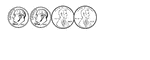22 Cents

Groups of change with totals from 1 to 100 cents using the least amount of coins.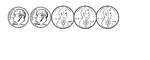23 Cents

Groups of change with totals from 1 to 100 cents using the least amount of coins.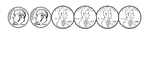24 Cents

Groups of change with totals from 1 to 100 cents using the least amount of coins.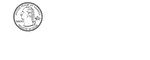25 Cents

Groups of change with totals from 1 to 100 cents using the least amount of coins.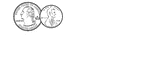26 Cents

Groups of change with totals from 1 to 100 cents using the least amount of coins.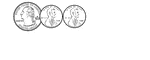27 Cents

Groups of change with totals from 1 to 100 cents using the least amount of coins.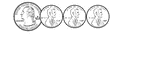28 Cents

Groups of change with totals from 1 to 100 cents using the least amount of coins.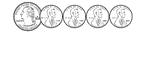29 Cents

Groups of change with totals from 1 to 100 cents using the least amount of coins.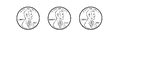3 Cents

Groups of change with totals from 1 to 100 cents using the least amount of coins.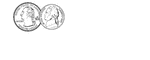30 Cents

Groups of change with totals from 1 to 100 cents using the least amount of coins.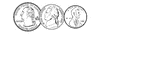31 Cents

Groups of change with totals from 1 to 100 cents using the least amount of coins.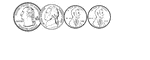32 Cents

Groups of change with totals from 1 to 100 cents using the least amount of coins.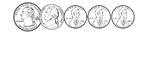33 Cents

Groups of change with totals from 1 to 100 cents using the least amount of coins.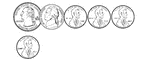34 Cents

Groups of change with totals from 1 to 100 cents using the least amount of coins.35 Cents

Groups of change with totals from 1 to 100 cents using the least amount of coins.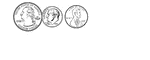36 Cents

Groups of change with totals from 1 to 100 cents using the least amount of coins.37 Cents

Groups of change with totals from 1 to 100 cents using the least amount of coins.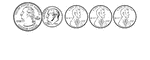38 Cents

Groups of change with totals from 1 to 100 cents using the least amount of coins.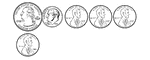39 Cents

Groups of change with totals from 1 to 100 cents using the least amount of coins.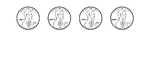4 Cents

Groups of change with totals from 1 to 100 cents using the least amount of coins.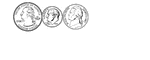40 Cents

Groups of change with totals from 1 to 100 cents using the least amount of coins.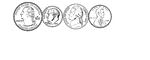41 Cents

Groups of change with totals from 1 to 100 cents using the least amount of coins.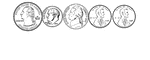42 Cents

Groups of change with totals from 1 to 100 cents using the least amount of coins.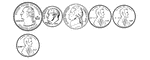43 Cents

Groups of change with totals from 1 to 100 cents using the least amount of coins.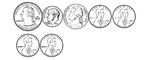44 Cents

Groups of change with totals from 1 to 100 cents using the least amount of coins.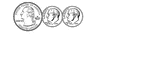45 Cents

Groups of change with totals from 1 to 100 cents using the least amount of coins.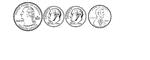46 Cents

Groups of change with totals from 1 to 100 cents using the least amount of coins.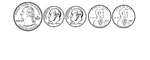47 Cents

Groups of change with totals from 1 to 100 cents using the least amount of coins.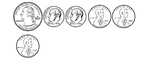48 Cents

Groups of change with totals from 1 to 100 cents using the least amount of coins.49 Cents

Groups of change with totals from 1 to 100 cents using the least amount of coins.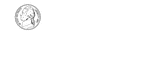5 Cents

Groups of change with totals from 1 to 100 cents using the least amount of coins.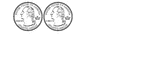50 Cents

Groups of change with totals from 1 to 100 cents using the least amount of coins.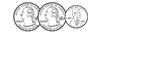51 Cents

Groups of change with totals from 1 to 100 cents using the least amount of coins.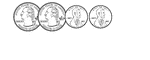52 Cents

Groups of change with totals from 1 to 100 cents using the least amount of coins.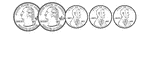53 Cents

Groups of change with totals from 1 to 100 cents using the least amount of coins.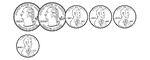54 Cents

Groups of change with totals from 1 to 100 cents using the least amount of coins.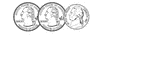55 Cents

Groups of change with totals from 1 to 100 cents using the least amount of coins.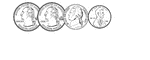56 Cents

Groups of change with totals from 1 to 100 cents using the least amount of coins.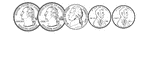57 Cents

Groups of change with totals from 1 to 100 cents using the least amount of coins.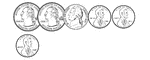58 Cents

Groups of change with totals from 1 to 100 cents using the least amount of coins.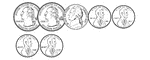59 Cents

Groups of change with totals from 1 to 100 cents using the least amount of coins.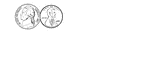6 Cents

Groups of change with totals from 1 to 100 cents using the least amount of coins.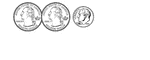60 Cents

Groups of change with totals from 1 to 100 cents using the least amount of coins.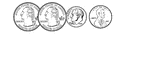61 Cents

Groups of change with totals from 1 to 100 cents using the least amount of coins.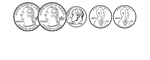62 Cents

Groups of change with totals from 1 to 100 cents using the least amount of coins.